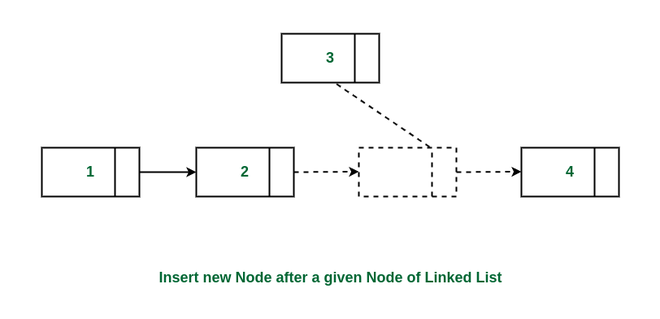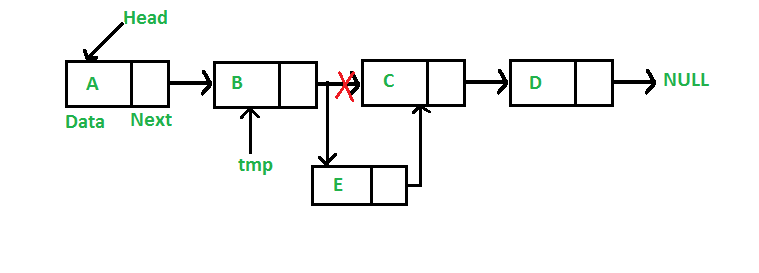Open in App
Not now

# Insert a Node after a given Node in Linked List

• Last Updated : 19 Oct, 2022

Like arrays, Linked List is a linear data structure. Unlike arrays, linked list elements are not stored at a contiguous location; the elements are linked using pointers. They include a series of connected nodes. Here, each node stores the data and the address of the next node.

Given a linked list, the task is to insert a new node after a given node of the linked list.Insert new node after a given node in linked list

Example:

List = 1->2->4->5, Insert a node with value 3 after the node with value 2.
Output list will be: 1->2->3->4->5

Consider the following representations of the linked list.

## C++

```// A linked list node
class Node {
public:
int data;
Node* next;
};```

## C

```// A linked list node
struct Node {
int data;
struct Node* next;
};```

## Java

```// Linked List Class

/* Node Class */
class Node {
int data;
Node next;

// Constructor to create a new node
Node(int d)
{
data = d;
next = null;
}
}
}```

## Python3

```# Node class
class Node:

# Function to initialize the node object
def __init__(self, data):
self.data = data  # Assign data
self.next = None  # Initialize next as null

# Function to initialize the Linked List object
def __init__(self):
```

## C#

```/* Linked list Node*/
public class Node {
public int data;
public Node next;
public Node(int d)
{
data = d;
next = null;
}
}```

## Javascript

```<script>

/* Node Class */
class Node {

// Constructor to create a new node
constructor(d) {
this.data = d;
this.next = null;
}
}
// This code is contributed by todaysgaurav
</script>
```

Approach: Follow the below steps for inserting a node after a given node:

• Firstly, check if the given previous node is NULL or not.
• Then, allocate a new node (say temp) and
• Assign the data to temp.
• And then make the next of temp as the next of the previous node.
• Finally, move the next of the previous node to point to temp.

Follow the below image for a better understanding.How to insert a new node after given node in linked list

Below is the implementation of the approach.

## C++

```// Given a node prev_node, insert a
// new node after the given
// prev_node
void insertAfter(Node* prev_node, int new_data)
{
// 1. Check if the given prev_node is NULL
if (prev_node == NULL) {
cout << "The given previous node cannot be NULL";
return;
}

// 2. Allocate new node
Node* new_node = new Node();

// 3. Put in the data
new_node->data = new_data;

// 4. Make next of new node as
// next of prev_node
new_node->next = prev_node->next;

// 5. move the next of prev_node
// as new_node
prev_node->next = new_node;
}```

## C

```/* Given a node prev_node, insert a new node after the given
prev_node */
void insertAfter(struct Node* prev_node, int new_data)
{
/*1. check if the given prev_node is NULL */
if (prev_node == NULL) {
printf("the given previous node cannot be NULL");
return;
}

/* 2. allocate new node */
struct Node* new_node
= (struct Node*)malloc(sizeof(struct Node));

/* 3. put in the data */
new_node->data = new_data;

/* 4. Make next of new node as next of prev_node */
new_node->next = prev_node->next;

/* 5. move the next of prev_node as new_node */
prev_node->next = new_node;
}```

## Java

```/* This function is in LinkedList class.
Inserts a new node after the given prev_node. This method is
defined inside LinkedList class shown above */
public void insertAfter(Node prev_node, int new_data)
{
/* 1. Check if the given Node is null */
if (prev_node == null) {
System.out.println(
"The given previous node cannot be null");
return;
}

/* 2. Allocate the Node &
3. Put in the data*/
Node new_node = new Node(new_data);

/* 4. Make next of new Node as next of prev_node */
new_node.next = prev_node.next;

/* 5. make next of prev_node as new_node */
prev_node.next = new_node;
}```

## Python3

```# This function is in LinkedList class.
# Inserts a new node after the given prev_node. This method is
# defined inside LinkedList class shown above */
def insertAfter(self, prev_node, new_data):

# 1. check if the given prev_node exists
if prev_node is None:
print("The given previous node must inLinkedList.")
return

# 2. Create new node &
# 3. Put in the data
new_node = Node(new_data)

# 4. Make next of new Node as next of prev_node
new_node.next = prev_node.next

# 5. make next of prev_node as new_node
prev_node.next = new_node
```

## C#

```/* Inserts a new node after the given prev_node. */
public void insertAfter(Node prev_node, int new_data)
{
/* 1. Check if the given Node is null */
if (prev_node == null) {
Console.WriteLine("The given previous node"
+ " cannot be null");
return;
}

/* 2 & 3: Allocate the Node &
Put in the data*/
Node new_node = new Node(new_data);

/* 4. Make next of new Node as
next of prev_node */
new_node.next = prev_node.next;

/* 5. make next of prev_node
as new_node */
prev_node.next = new_node;
}```

## Javascript

```<script>

/* This function is in LinkedList class.
Inserts a new node after the given prev_node. This method is
defined inside LinkedList class shown above */
function insertAfter(prev_node, new_data)
{

/* 1. Check if the given Node is null */
if (prev_node == null)
{
document.write("The given previous node cannot be null");
return;
}

/* 2. Allocate the Node &
3. Put in the data*/
var new_node = new Node(new_data);

/* 4. Make next of new Node as next of prev_node */
new_node.next = prev_node.next;

/* 5. make next of prev_node as new_node */
prev_node.next = new_node;
}

// This code is contributed by aashish1995

</script>```

Time Complexity: O(1)
Auxiliary Space: O(1)

My Personal Notes arrow_drop_up
Related Articles